# Texas Go Math Grade 2 Lesson 14.1 Answer Key Two-Dimensional Shapes

Refer to our Texas Go Math Grade 2 Answer Key Pdf to score good marks in the exams. Test yourself by practicing the problems from Texas Go Math Grade 2 Lesson 14.1 Answer Key Two-Dimensional Shapes.

## Texas Go Math Grade 2 Lesson 14.1 Answer Key Two-Dimensional Shapes

Explore

Use a ruler. Draw a shape with 3 straight sides. Then draw a shape with 4 straight sides.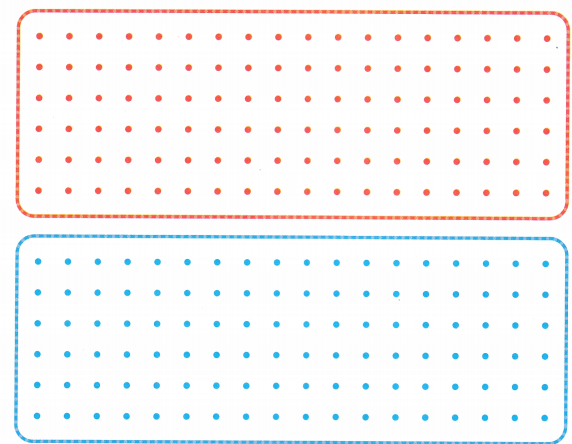FOR THE TEACHER • Have children use rulers as straight edges for drawing the sides of shapes. Have children draw a two-dimensional shape with 3 sides and then a two-dimensional shape with 4 sides.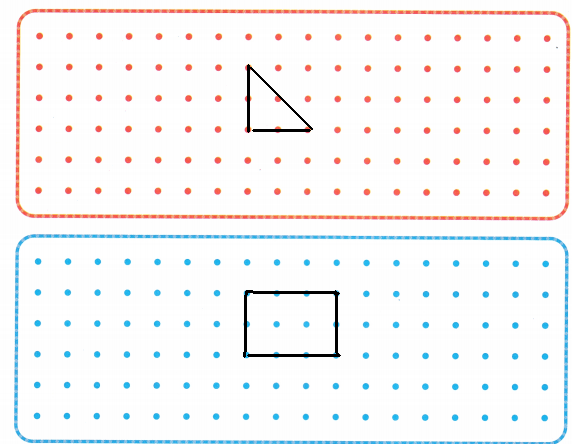Explanation:
In the above image we can observe two shapes. The shape with 3 straight sides is called as triangle. The shape with 4 straight sides is called as quadrilateral.

Math Talk
Mathematical Processes

Describe how your shapes are different from the shapes a classmate drew.The classmate drawn a triangle and quadrilateral. I drawn pentagon and hexagon. So, the shapes are different.

Model and Draw

You can count sides and vertices to name two-dimensional shapes.
How many sides and vertices does each shape have?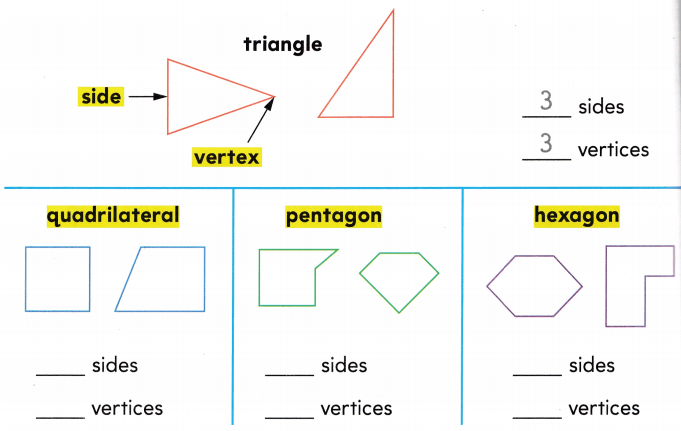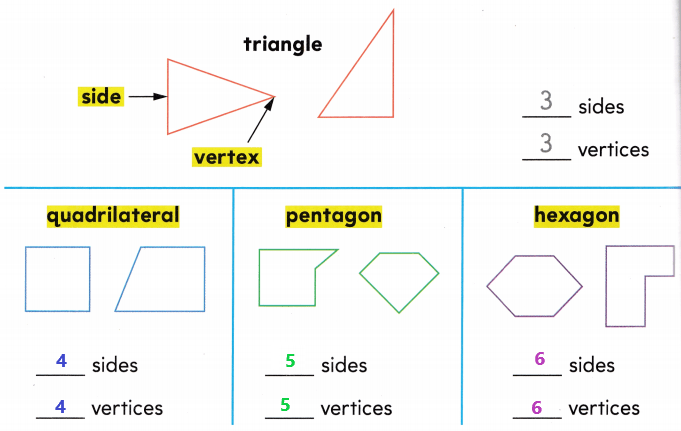Explanation:
A polygon with 3 sides is called as triangle. This polygon has 3 vertices.
A polygon with 4 sides is called as quadrilateral. This polygon has 4 vertices.
A polygon with 5 sides is called as pentagon. This polygon has 5 vertices.
A polygon with 6 sides is called as hexagon. This polygon has 6 vertices.

Share and Show

Write the number of sides and the number of vertices.

Question 1.
triangle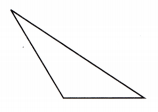__________ sides
__________ vertices
A triangle has 3 sides and 3 vertices.
Explanation:
A triangle is a polygon with three edges and three vertices. It is one of the basic shapes in geometry.

Question 2.
hexagon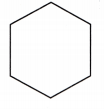__________ sides
__________ vertices
A hexagon has 6 sides and 6 vertices.
Explanation:
In geometry, a hexagon is a six-sided polygon or 6-gon.

Question 3.
pentagon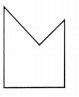__________ sides
__________ vertices
A pentagon has 5 sides and 5 vertices.
Explanation:
In geometry, a pentagon is any five-sided polygon or 5-gon.

Problem Solving

Write the number of sides and the number of vertices. Then write the name of the shape.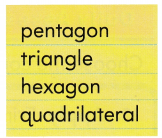Question 4.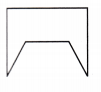__________ sides
__________ vertices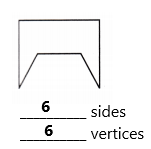The name of the above shape is Hexagon.
Explanation:
In the above image we can observe one polygon. The polygon has 6 sides and 6 vertices. The name of the above shape is Hexagon. In geometry, a hexagon is a six-sided polygon or 6-gon.

Question 5.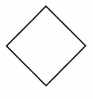__________ sides
__________ vertices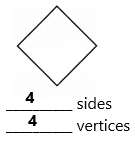The name of the above shape is quadrilateral.
Explanation:
In the above image we can observe one polygon. A quadrilateral is a polygon with four edges (sides) and four vertices (corners).

Question 6.__________ sides
__________ vertices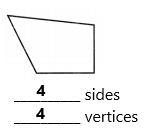The name of the above shape is quadrilateral.
Explanation:
In the above image we can observe one polygon. A quadrilateral is a polygon with four edges (sides) and four vertices (corners).

Question 7.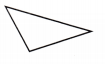__________ sides
__________ vertices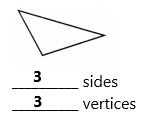The name of the above shape is triangle.
Explanation:
In the above image we can observe one polygon. A triangle is a polygon with three edges and three vertices. It is one of the basic shapes in geometry.

Question 8.__________ sides
__________ verticesThe name of the above shape is pentagon.
Explanation:
In the above image we can observe one polygon. A polygon has 5 sides and 5 vertices. The name of the above shape is pentagon.

Question 9.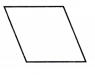__________ sides
__________ vertices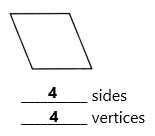The name of the above shape is quadrilateral.
Explanation:
In the above image we can observe one polygon. A quadrilateral is a polygon with four edges (sides) and four vertices (corners).

Question 10.
H.O.T. Multi-Step Alex draws a hexagon and two pentagons. How many sides does Alex draw altogether?________ sides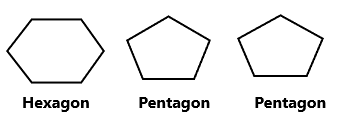6 sides + 5 sides + 5 sides = 16 sides
Alex drawn 16 sides altogether.
Explanation:
Alex drawn a hexagon and two pentagons. A hexagon has 6 sides and 6 vertices. A pentagon has 5 sides and 5 vertices. Add 6 sides with 5 sides and 5 sides the sum is 16 sides. So, Alex drawn 16 sides altogether.

Question 11.
H.O.T. Lydia draws a shape that has 4 vertices. It is not a square. It is not a rectangle. Draw a shape that could be Lydia’s shape.Explanation:
Lydia drawn a shape that has 4 vertices. It is not a square. It is not a rectangle. Lydia’s shape of the polygon is quadrilateral.

Question 12.
Apply Erin sees this shape. What ¡s the shape?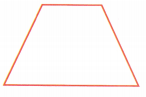(A) pentagon
(B) triangle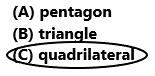So, option C is correct.
Explanation:
Erin saw the above shape. The shape of the above polygon is quadrilateral. A quadrilateral is a polygon with four edges (sides) and four vertices (corners). So, draw a circle for option C.

Question 13.
How many sides does this shape have?_________ sides
The above shape have 5 sides.
Explanation:
In the above image we can observe one polygon. The name of the above polygon is pentagon. A pentagon has 5 sides and 5 vertices.

Question 14.
How many vertices does this shape have?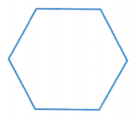_________ vertices
The above shape has 6 vertices.
Explanation:
In the above image we can observe one polygon. The name of the above polygon is hexagon. A hexagon has 6 sides and 6 vertices.

Question 15.
Texas Test Prep What is the shape of this sign?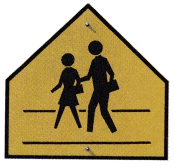(B) hexagon
(C) pentagon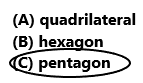Option C is correct.
Explanation:
The shape of the above sign is pentagon. Pentagon has 5 sides and 5 vertices. So, draw a circle for option C.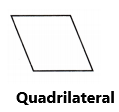Explanation:
A quadrilateral is a polygon with four edges (sides) and four vertices (corners).

### Texas Go Math Grade 2 Lesson 14.1 Homework and Practice Answer Key

Write the number of sides and the number of vertices.

Question 1.
triangle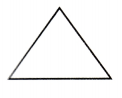__________ sides
__________ vertices
A triangle has 3 sides and 3 vertices.
Explanation:
A triangle is a polygon with three edges and three vertices. It is one of the basic shapes in geometry.

Question 2.__________ sides
__________ vertices
A quadrilateral has 4 sides and 4 vertices.
Explanation:
In the above image we can observe one polygon. A quadrilateral is a polygon with four edges (sides) and four vertices (corners).

Question 3
pentagon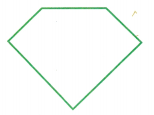__________ sides
__________ vertices
A pentagon has 5 sides and 5 vertices.
Explanation:
In the above image we can observe one polygon. A pentagon has 5 sides and 5 vertices.

Write the number of sides and the number of vertices. Then write the name of the shape.

Question 4.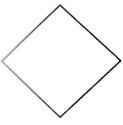__________ sides
__________ vertices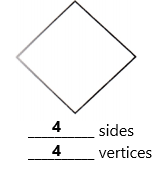The name of the above shape is quadrilateral.
Explanation:
In the above image we can observe one polygon. The name of the above shape is  quadrilateral. A quadrilateral is a polygon with four edges (sides) and four vertices (corners).

Question 5.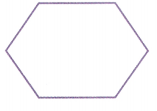__________ sides
__________ verticesThe above shape is hexagon.
Explanation:
In the above image we can observe one polygon. The name of the above polygon is hexagon. A hexagon has 6 sides and 6 vertices.

Question 6.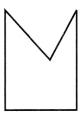__________ sides
__________ verticesThe above shape is pentagon.
Explanation:
In the above image we can observe one polygon. The name of the above polygon is pentagon. A pentagon has 5 sides and 5 vertices.

Problem Solving

Question 7.
Multi-Step Brittany draws a pentagon and two triangles. How many sides does Brittany draw altogether?
_________ sides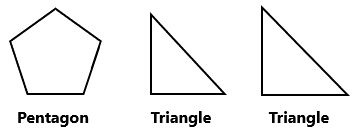5 sides + 3 sides + 3 sides = 11 sides
Brittany drawn 11 sides altogether.
Explanation:
Brittany drawn a pentagon and two triangles. A pentagon has 5 sides and 5 vertices. A triangle has 3 sides and 3 vertices. Add 5 sides with 3 sides and 3 sides the sum is 11 sides. Brittany drawn 11 sides altogether.

Lesson Check

Question 8.
What is the shape of this plate?(A) pentagon
(C) hexagonOption C is correct.
Explanation:
The shape of the above plate is hexagon. Hexagon has 6 sides and 6 vertices. So, draw a circle for option C.

Question 9.
Ben’s favorite shape has 5 sides and 5 vertices. What is Ben’s favorite shape?
(A) triangle
(B) pentagon
(C) hexagonOption B is correct.
Explanation:
Ben’s favorite shape has 5 sides and 5 vertices. So, Ben’s favorite shape is pentagon. Draw a circle for option B.

Question 10.
Lynn drew this shape. What is the name this shape?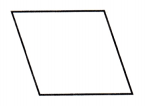(B) triangle
(C) pentagonoption A is correct.
Explanation:
Lynn drawn the above shape. The name of the above shape is quadrilateral. A quadrilateral has 4 sides and 4 vertices. So, draw a circle for option A.

Question 11.
Matthew sees this shape. What is the shape?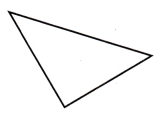(A) hexagon
(B) triangle